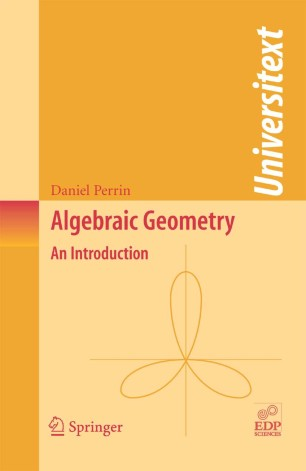# Algebraic Geometry

## An Introduction

• Daniel PerrinTextbook

Part of the Universitext book series (UTX)

1. Front Matter
Pages I-XX
2. Pages 9-24
3. Pages 25-36
4. Pages 37-68
5. Pages 69-86
6. Pages 87-99
7. Pages 101-112
8. Pages 113-129
9. Pages 149-171
10. Pages 173-195
11. Back Matter
Pages 199-263

### Introduction

Aimed primarily at graduate students and beginning researchers, this book provides an introduction to algebraic geometry that is particularly suitable for those with no previous contact with the subject and assumes only the standard background of undergraduate algebra. It is developed from a masters course given at the Université Paris-Sud, Orsay, and focusses on projective algebraic geometry over an algebraically closed base field.

The book starts with easily-formulated problems with non-trivial solutions – for example, Bézout’s theorem and the problem of rational curves – and uses these problems to introduce the fundamental tools of modern algebraic geometry: dimension; singularities; sheaves; varieties; and cohomology. The treatment uses as little commutative algebra as possible by quoting without proof (or proving only in special cases) theorems whose proof is not necessary in practice, the priority being to develop an understanding of the phenomena rather than a mastery of the technique. A range of exercises is provided for each topic discussed, and a selection of problems and exam papers are collected in an appendix to provide material for further study.

### Keywords

Algebra Arithmetic Riemann-Roch theorem algebraic geometry cohomology curves dimension geometry projective geometry proof sheaves singular points theorem

#### Authors and affiliations

• Daniel Perrin
• 1
1. 1.Département de MathématiquesBât. 425 Faculté des Sciences d'Orsay Université Paris-Sud 11Orsay CedexFrance

Industry Sectors